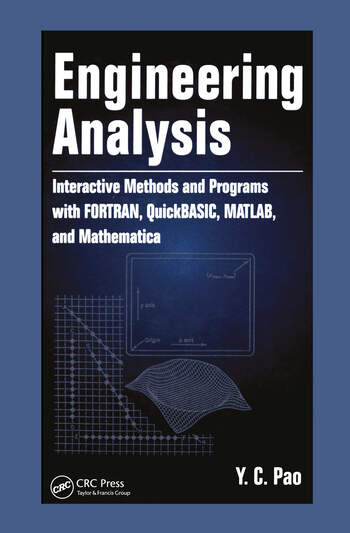Engineering Analysis: Interactive Methods and Programs with FORTRAN, QuickBASIC, MATLAB, and Mathematica

1st Edition

Yen-Ching Pao

CRC Press
Published August 28, 1998
Reference - 384 Pages
ISBN 9780849320163 - CAT# 2016

For Instructors Request Inspection Copy

USD\$185.00

Currently out of stock
FREE Standard Shipping!

Preview

Summary

This book provides a concise introduction to numerical concepts in engineering analysis, using FORTRAN, QuickBASIC, MATLAB, and Mathematica to illustrate the examples. Discussions include:
• matrix algebra and analysis
• solution of matrix equations
• methods of curve fit
• methods for finding the roots of polynomials and transcendental equations
• finite differences and methods for interpolation and numerical differentiation
• numerical computation of single and double integrals
• numerical solution of ordinary differential equations
Engineering Analysis:
• teaches readers to become proficient in FORTRAN or QuickBASIC programming to solve engineering problems
• provides an introduction to MATLAB and Mathematica, enabling readers to write supplementary m-files for MATLAB and toolkits for Mathematica using C-like commands
The book emphasizes interactive operation in developing computer programs throughout, enabling the values of the parameters involved in the problem to be entered by the user of the program via a keyboard.
In introducing each numerical method, Engineering Analysis gives minimum mathematical derivations but provides a thorough explanation of computational procedures to solve a specific problem. It serves as an exceptional text for self-study as well as resource for general reference.
• Instructors

We provide complimentary e-inspection copies of primary textbooks to instructors considering our books for course adoption.